Bisector of an angle

Chapter 14 Class 6 Practical Geometry
Concept wise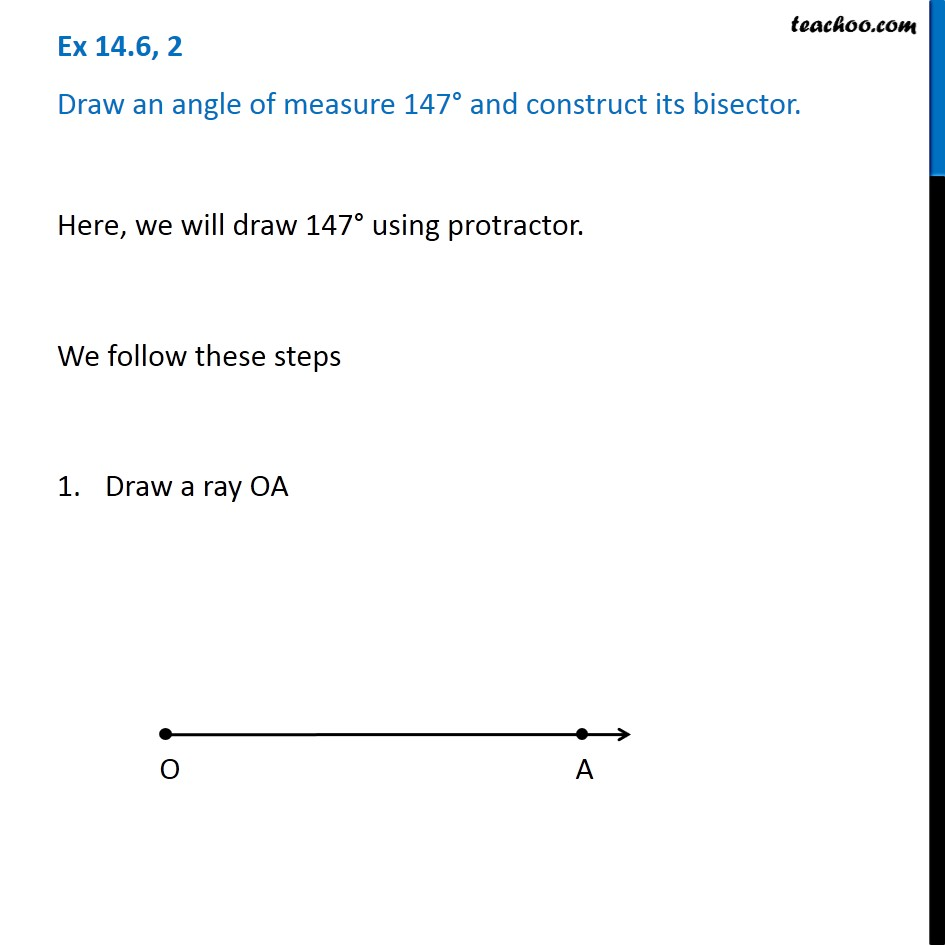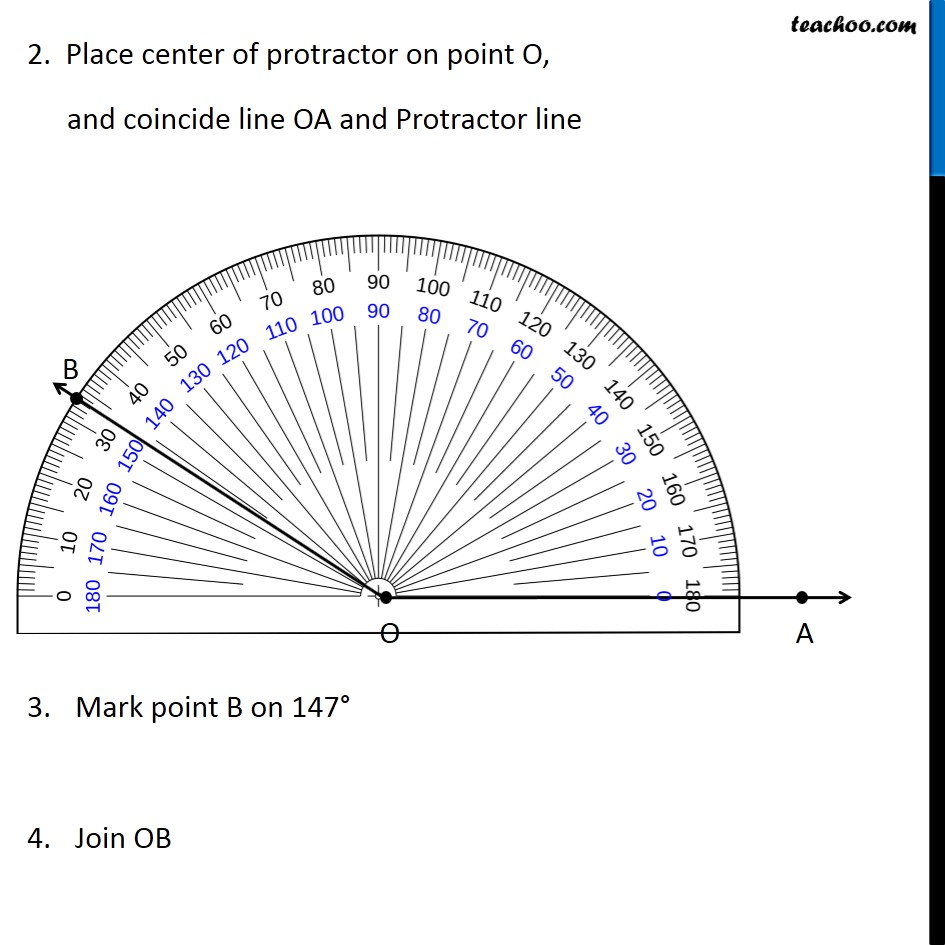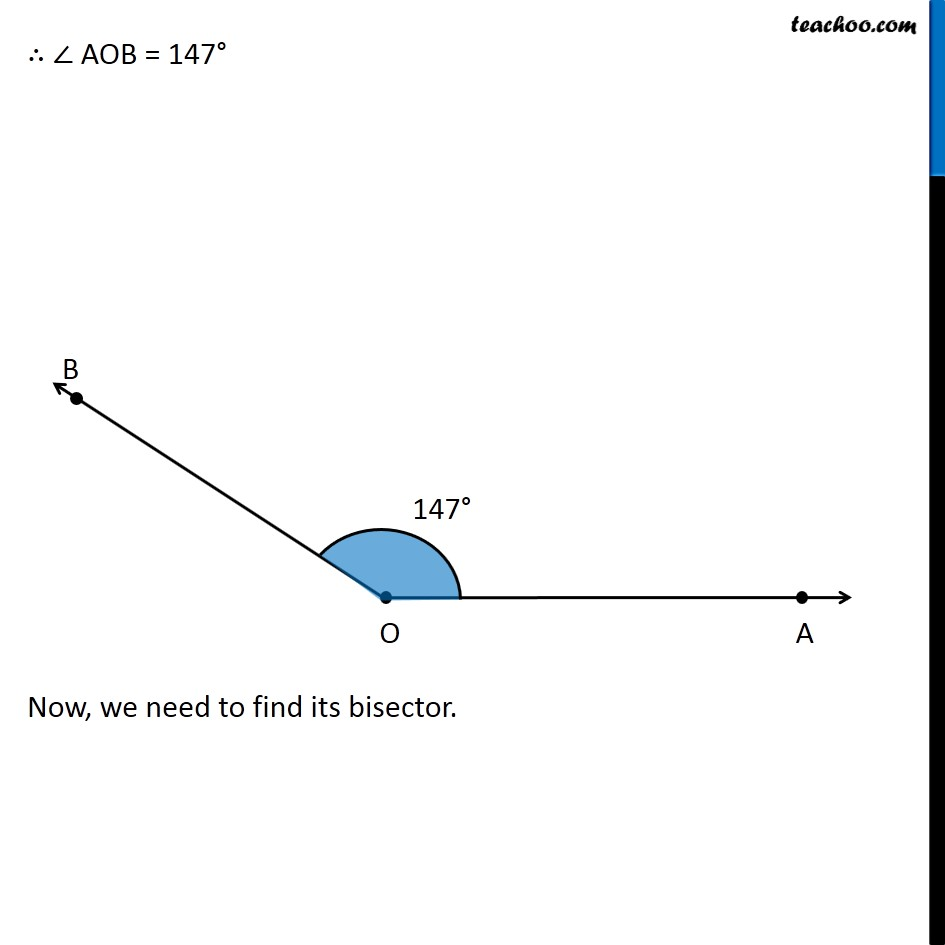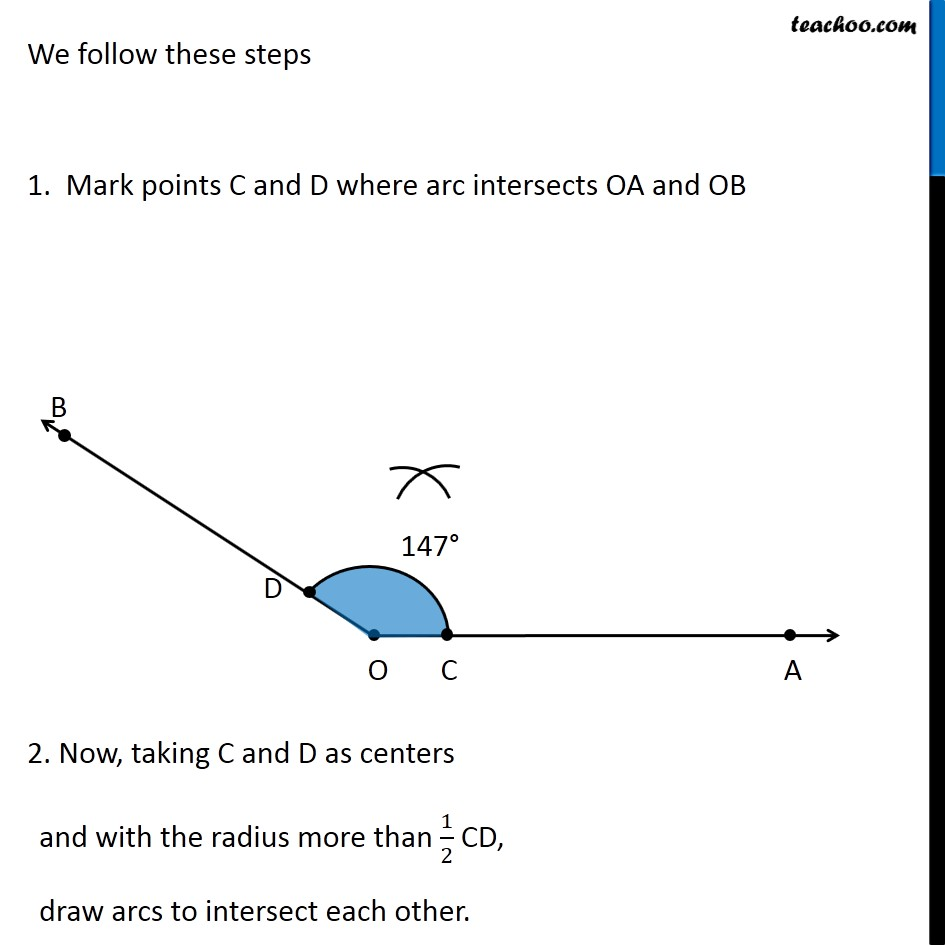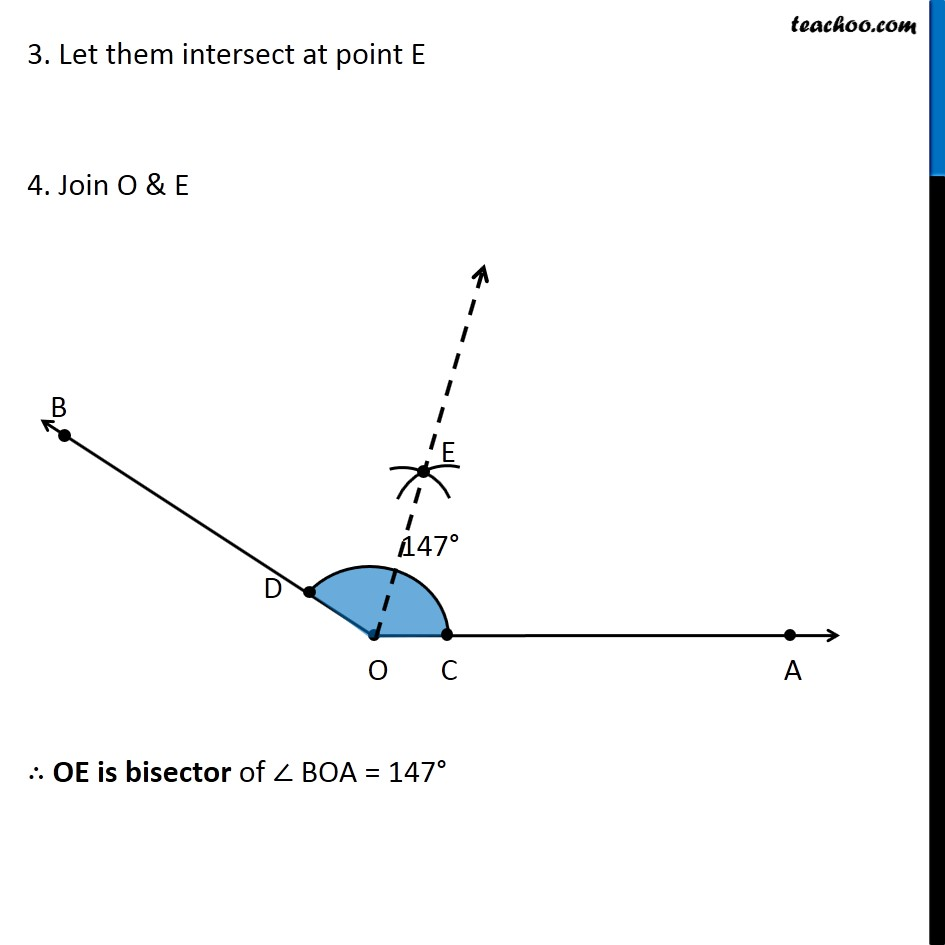Introducing your new favourite teacher - Teachoo Black, at only ₹83 per month

### Transcript

Ex 14.6, 2 Draw an angle of measure 147° and construct its bisector. Here, we will draw 147° using protractor. We follow these steps Draw a ray OA 2. Place center of protractor on point O, and coincide line OA and Protractor line Mark point B on 147° Join OB ∴ ∠ AOB = 147° Now, we need to find its bisector. We follow these steps 1. Mark points C and D where arc intersects OA and OB 2. Now, taking C and D as centers and with the radius more than 1/2 CD, draw arcs to intersect each other. 3. Let them intersect at point E 4. Join O & E ∴ OE is bisector of ∠ BOA = 147°